﻿ 基于Copula函数的学生成绩极值与均值的相关性研究 The Study of the Correlation between Extreme Value and Mean Value of the Student Achievement Based on Copula Function

Vol.06 No.04(2017), Article ID:21399,7 pages
10.12677/AAM.2017.64061

The Study of the Correlation between Extreme Value and Mean Value of the Student Achievement Based on Copula Function

Hong Chang, Fuxia Xu*

School of Science, Tianjin Polytechnic University, Tianjin

*通讯作者。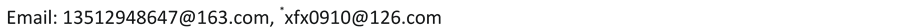Received: Jun. 25th, 2017; accepted: Jul. 15th, 2017; published: Jul. 18th, 2017ABSTRACT

Based on the semiparametric estimation method of nonparametric kernel density and the square and Euclidean distance with empirical Copula functions, the Gumbel Copula model is established for the extreme and mean value of the student achievement. We rely on the Gumbel Copula function to investigate the correlation between the highest score, the lowest score and the average score. It turns out that the Kendall rank correlation coefficient and the upper tail correlation coefficient are slightly higher than the highest points.

Keywords:Copula Function, Kerneldensity Estimation, Semiparametric Estimation, Rank Correlation, Tail Correlation1. 引言

2. 问题的分析Table 1. The highest score, the lowest score and the average score of points in some classesTable 2. Skewness, kurtosis and normality test resultsFigure 1. Frequency distribution histogram

3. Copula函数模型的建立

3.1. 参数估计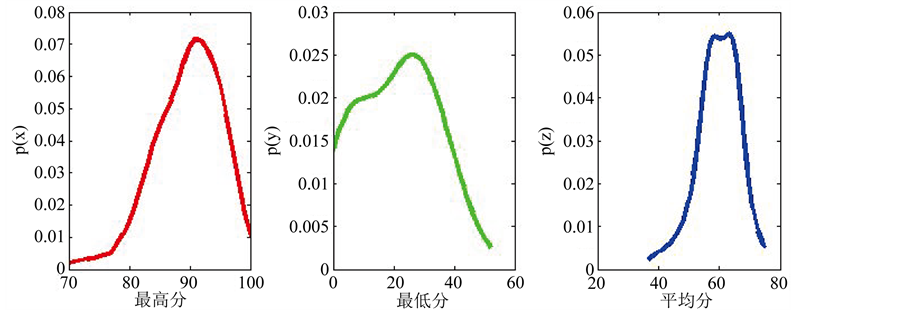Figure 2. Kernel density estimation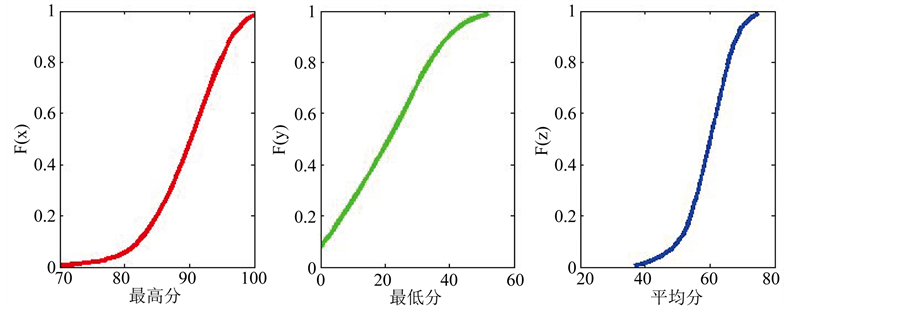Figure 3. Estimation of nuclear distribution

3.2. 模型的选取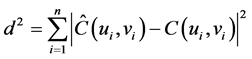(1)Table 3. Parameter estimation results of Copula functions with the highest score and the average scoreTable 4. Parameter estimation results of Copula functions with the lowest score and the average scoreTable 5. Square Euclidean distance of Copula functions with the highest score and the average scoreTable 6. Square Euclidean distance of Copula functions with the lowest score and the average score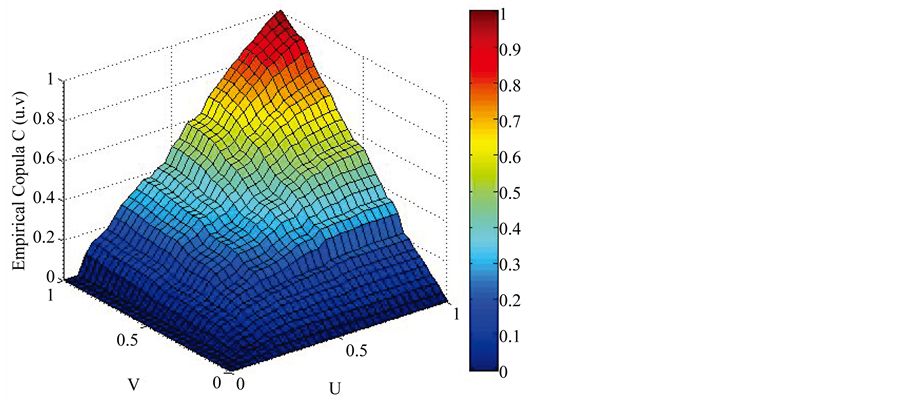Figure 4. The distribution function of Empirical Copula(2)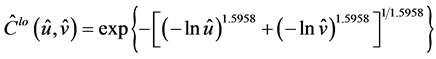(3)

4. 相关性分析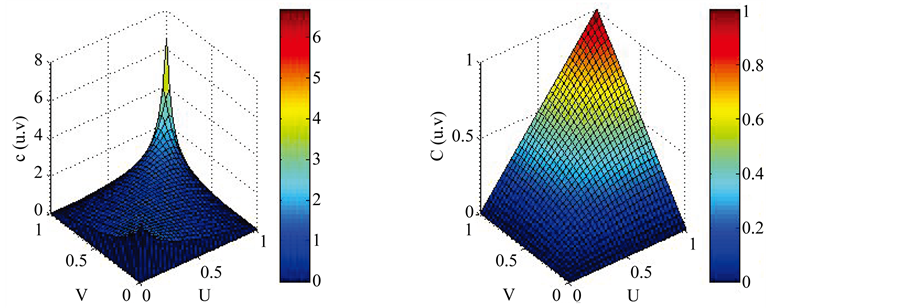Figure 5. The density function graph and distribution function graph of Gumbel-Copula between the highest score and average scoreFigure 6. The density function graph and distribution function graph of Gumbel-Copula between the lowest score and average scoreTable 7. Correlation coefficient based on Gumbel Copula

5. 结论

The Study of the Correlation between Extreme Value and Mean Value of the Student Achievement Based on Copula Function[J]. 应用数学进展, 2017, 06(04): 508-514. http://dx.doi.org/10.12677/AAM.2017.64061

1. 1. 张晓宇, 徐付霞. 基于Copula理论的学生成绩平均值和中位数的分布特征研究[J]. 大学数学, 2016, 32(1): 56-60.

2. 2. 梁冯珍, 史道济. 离散型最小和最大次序统计量相关性研究[J]. 应用概率统计, 2008, 24(4): 381-387.

3. 3. 徐付霞, 董永权. 泥石流地貌要素的极值相关性[J]. 系统工程理论与实践, 2009, 29(2): 180-185.

4. 4. 李霞. COPULA方法及其应用[M]. 北京: 经济管理出版社, 2014.

5. 5. 谢中华. MATLAB统计分析与应用[M]. 北京: 北京航空航天大学出版社, 2010.

6. 6. 尹向飞. 基于混合正态分布的大学生考试成绩分布的拟合[J]. 统计与决策, 2007(8): 133-135.

7. 7. 吴喜之, 赵博娟. 非参数统计[M]. 第3版. 北京: 中国统计出版社, 2009: 175-180.

8. 8. Nelsen, R.B. (2006) An Introduction to Copulas. 2nd Edition, Springer, New York.

9. 9. Juri, A. and Wüthrich, M.V. (2002) Copula Convergence Theorems for Tail Events. Insurance Mathematics and Economics, 30, 405-420.

10. 10. 李悦, 程希骏. 上证指数和恒生指数的copula尾部相关性分析[J]. 系统工程, 2006, 24(5): 88-92.